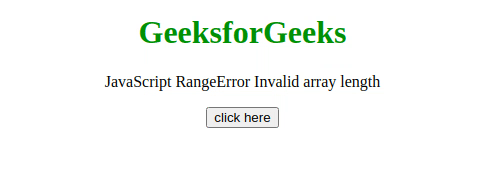# JavaScript RangeError – Invalid array length

This JavaScript exception Invalid array length occurs when creating an Array or an ArrayBuffer of the array length either negative or greater than or equal to 232. It can also occur if the length property is set manually to a value either negative or greater than equal to 232.

Message:

```RangeError: Array length must be a finite positive integer (Edge)
RangeError: invalid array length (Firefox)
RangeError: Invalid array length (Chrome)
RangeError: Invalid array buffer length (Chrome)
```

Error Type:

```RangeError
```

Cause of the error: The length of an Array or an ArrayBuffer can only be represented by an unsigned 32-bit integer, which only stores values ranging from 0 to 232-1. While creating an Array or an ArrayBuffer, if the array length is either negative or greater than or equal to 232  then this error occurs.

Example 1: In this example, the length property is set to 6, which is a valid value, therefore no error occurred.

## HTML

 ` ` `<``html``> ` `    ``<``head``> ` `        ``<``script` `src``= ` `"https://code.jquery.com/jquery-3.5.0.js"``> ` `        `` ` `    `` ` `    ``<``body` `style``=``"text-align: center;"``> ` `        ``<``h1` `style``=``"color: green;"``> ` `            ``GeeksforGeeks ` `        `` ` ` `  `        ``<``p``> ` `            ``JavaScript RangeError  ` `            ``Invalid array length ` `        `` ` ` `  `        ``<``button` `onclick``=``"Geeks();"``> ` `            ``click here ` `        `` ` `        ``<``p` `id``=``"GFG_DOWN"``> ` ` `  `        ``<``script``> ` `            ``var el_down = document.getElementById("GFG_DOWN"); ` `            ``function Geeks() { ` `                ``try { ` `                    ``let a = []; ` `                    ``a.length = 6; ` `                    ``el_down.innerHTML =  ` `                      ``"'Invalid array length' "+ ` `                      ``"error has not occurred"; ` `                ``} catch (e) { ` `                   `  `                    ``// Show the error in console ` `                    ``console.log(e); ` `                    ``el_down.innerHTML =  ` `                      ``"'Invalid array length' "+ ` `                      ``"error has occurred"; ` `                ``} ` `            ``} ` `        `` ` `    `` ` ` `

Output:Example 2: In this example, the length property is set to -1, which is an invalid value, therefore the error has occurred.

## HTML

 ` ` `<``html``> ` `    ``<``head``> ` `        ``<``script` `src``= ` `"https://code.jquery.com/jquery-3.5.0.js"``> ` `        `` ` `    `` ` `    ``<``body` `style``=``"text-align: center;"``> ` `        ``<``h1` `style``=``"color: green;"``> ` `            ``GeeksforGeeks ` `        `` ` ` `  `        ``<``p``> ` `            ``JavaScript RangeError  ` `            ``Invalid array length ` `        `` ` ` `  `        ``<``button` `onclick``=``"Geeks();"``> ` `            ``click here ` `        `` ` `        ``<``p` `id``=``"GFG_DOWN"``> ` ` `  `        ``<``script``> ` `            ``var el_down = document.getElementById("GFG_DOWN"); ` `            ``function Geeks() { ` `                ``try { ` `                    ``let a = []; ` `                    ``a.length = -1; ` `                    ``el_down.innerHTML =  ` `                      ``"'Invalid array length' "+ ` `                      ``"error has not occurred"; ` `                ``} catch (e) { ` `                   `  `                    ``// Show the error in console ` `                    ``console.log(e); ` `                    ``el_down.innerHTML =  ` `                      ``"'Invalid array length' "+ ` `                      ``"error has occurred"; ` `                ``} ` `            ``} ` `        `` ` `    `` ` ` `

Output:My Personal Notes arrow_drop_upCheck out this Author's contributed articles.

If you like GeeksforGeeks and would like to contribute, you can also write an article using contribute.geeksforgeeks.org or mail your article to contribute@geeksforgeeks.org. See your article appearing on the GeeksforGeeks main page and help other Geeks.

Please Improve this article if you find anything incorrect by clicking on the "Improve Article" button below.

Article Tags :

Be the First to upvote.

Please write to us at contribute@geeksforgeeks.org to report any issue with the above content.